Introduction

Adding and subtracting radicals is very similar to adding and subtracting like terms. The radical acts like the variable when adding like terms, and has to be exactly the same in order to add or subtract. For exampleis very similar to 3x + 5x. Where 3x + 5x = 8x, the radical addition is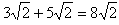.

Example 1 Simplify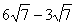.

Step 1. Verify the radicals are the same.

Both terms contain a radical of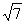.

Step 2. Combine like terms.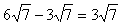Example 2 Simplify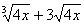.

Step 1. Verify the radicals are the same.

The radicals are not the same because one is a cube root and the other is a square root. Therefore this expression is in simplest form.